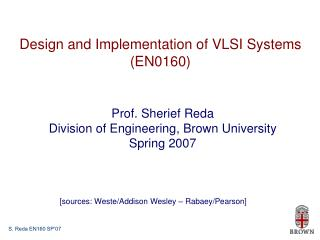Download PresentationDesign and Implementation of VLSI Systems (EN0160)

# Design and Implementation of VLSI Systems (EN0160) - PowerPoint PPT PresentationDownload Presentation## Design and Implementation of VLSI Systems (EN0160)

- - - - - - - - - - - - - - - - - - - - - - - - - - - E N D - - - - - - - - - - - - - - - - - - - - - - - - - - -
##### Presentation Transcript

1. Design and Implementation of VLSI Systems (EN0160) Prof. Sherief Reda Division of Engineering, Brown University Spring 2007 [sources: Weste/Addison Wesley – Rabaey/Pearson]

2. SPICE, a Simulation Program with Integrated Circuit Emphasis SPICE introduction SPICE card SPICE deck • We will use SmartSPICE by SimuCAD (http://www.engin.brown.edu/vpn)

3. SPICE is case insensitive Cards beginning with a dot (.) are control cards Cards beginning with a * are comment cards The last card must be .end Each card in the netlist must begin with a letter indicating its type SPICE Intro

4. Letter Circuit Element R Resistor C Capacitor L Inductor K Mutual Inductor V Independent voltage source I Independent current source M MOSFET D Diode Q Bipolar transistor W Lossy transmission line X Subcircuit E Voltage-controlled voltage source G Voltage-controlled current source H Current-controlled voltage source F Current-controlled current source SPICE circuit elements

5. Units

6. DC Source Vdd vdd gnd 2.5 Piecewise Linear Source Vin in gnd pwl 0ps 0 100ps 0 150ps 1.8 800ps 1.8 Pulsed Source Vck clk gnd PULSE 0 1.8 0ps 100ps 100ps 300ps 800ps Voltage sources (time, voltage) pairs

7. RC response *rc.sp .option post Vin in gnd pwl 0ps 0 100ps 0 150ps 1.8 800ps 1.8 R1 in out 2k C1 out gnd 100f .tran 20ps 800ps .plot v(in) v(out) .end Tutorial movie at http://ic.engin.brown.edu/classes/EN160S07/spice.avi

8. .option post .include 'tsmc-180.txt' .temp 70 .option scale=90n Vgs g gnd 0 Vds d gnd 0 M1 d g gnd gnd NMOS W=2 L=2 .dc Vds 0 1.8 0.05 sweep vgs 0 1.8 0.3 .plot i(m1) .end NMOS I-V characteristics Mname drain gate source body type W=<width> L=<length>

9. NMOS I-V characteristics

10. .option post .include 'tsmc-180.txt' .temp 70 .option scale=90n Vdd vdd gnd 1.8 M1 d g gnd gnd NMOS W=4 L=2 AS=20 PS=18 AD=20 PD=18 M2 d g vdd vdd PMOS W=8 L=2 AS=40 PS=26 AD=40 PD=26 Vgs g gnd PULSE 0 1.8 0ps 10ps 10ps 100ps 220ps .tran 20ps 440ps .end Inverter transient analysis for diffusion capacitance calculations

11. Inverter transient analysis bootstrapping

12. * delay measurement .param SUPPLY=1.8 .param H=4 .option scale=90nm .include 'tsmc-180.txt' .temp 70 .option post .global vdd gnd .subckt inv a y N=4 P=8 M1 y a gnd gnd NMOS W='N' L=2 M2 y a vdd vdd PMOS W='P' L=2 .ends Vdd vdd gnd 'SUPPLY' Vin a gnd PULSE 0 'SUPPLY' 0ps 100ps 100ps 500ps 1000ps X1 a b inv N=4 P=8 X2 b gnd inv N=16 P=32 .tran 1ps 1000ps .measure tpdr TRIG v(a) VAL='SUPPLY/2' FALL=1 TARG v(b) VAL='SUPPLY/2' rise=1 .end Ignoring diffusion capacitance!! Measuring propagation delay a

13. .option post .include 'tsmc-180.txt' .temp 70 .option scale=90n Vgs g gnd 0 Vds d gnd 1.8 M1 d g gnd gnd NMOS W=2 L=2 .dc Vgs 0 1.8 0.05 s .plot i(m1) .end Leakage current/threshold voltage

14. This lecture: SPICE tutorial. (you can find more in chapter 5) We finished chapters 1 and 2 and parts of chapter 3 (CMOS fabrication) Next time: Relevant parts from chapter 3 and Tanner L-Edit tutorial Please starting working on assignment 2 ASAP Summary